# Colleges with the lowest SAT scores in Georgia

Top 10 colleges in Georgia with the lowest SAT scores
Looking for the colleges with the lowest SAT scores in Georgia? Well you're in luck! We've compiled a national college database and have created a list of the top 10 universities with the lowest SAT scores in Georgia below. If you are not a good test taker or worried about your test scores, this list is for you. These are the schools whose applicants had the lowest average SAT scores in Georgia, which means that you can get into these colleges with a lower SAT score. We also include each college's ACT scores and acceptance rate so that you can see where you would have the easiest time getting in. Read on to find out more.

## Paine College SAT scores

The average SAT score for Paine College is 830.The average SAT score of 830 breaks down into:

• SAT math: 410

The average ACT score for Paine College is 16 and their acceptance rate is 32%.

## Middle Georgia State University SAT scores

The average SAT score for Middle Georgia State University is 935.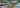The average SAT score of 935 breaks down into:

• SAT math: 460

The average ACT score for Middle Georgia State University is 19 and their acceptance rate is 94%.

## Savannah State University SAT scores

The average SAT score for Savannah State University is 940.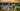The average SAT score of 940 breaks down into:

• SAT math: 460

The average ACT score for Savannah State University is 17 and their acceptance rate is 82.6%.

## Clayton State University SAT scores

The average SAT score for Clayton State University is 951.The average SAT score of 951 breaks down into:

• SAT math: 460

The average ACT score for Clayton State University is 20 and their acceptance rate is 48%.

## Life University SAT scores

The average SAT score for Life University is 970.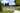The average SAT score of 970 breaks down into:

• SAT math: 480

The average ACT score for Life University is 19 and their acceptance rate is 99.3%.

## Columbus State University SAT scores

The average SAT score for Columbus State University is 978.The average SAT score of 978 breaks down into:

• SAT math: 480

The average ACT score for Columbus State University is 21 and their acceptance rate is 53.4%.

## University of West Georgia SAT scores

The average SAT score for University of West Georgia is 1000.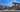The average SAT score of 1000 breaks down into:

• SAT math: 470

The average ACT score for University of West Georgia is 20 and their acceptance rate is 58.6%.

## Pfeiffer University SAT scores

The average SAT score for Pfeiffer University is 1010.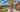The average SAT score of 1010 breaks down into:

• SAT math: 500

The average ACT score for Pfeiffer University is 19 and their acceptance rate is 66%.

## Georgia Gwinnett College SAT scores

The average SAT score for Georgia Gwinnett College is 1012.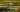The average SAT score of 1012 breaks down into:

• SAT math: 500

The average ACT score for Georgia Gwinnett College is 19 and their acceptance rate is 88%.

## Georgia South Western State University SAT scores

The average SAT score for Georgia South Western State University is 1041.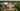The average SAT score of 1041 breaks down into:

• SAT math: 510